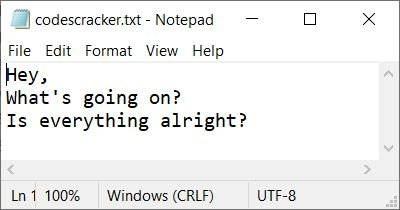# PHP fseek(): Move File Pointer

The PHP fseek() function is used when we need to move the file pointer to a particular position in the file. For example:

```<?php
\$file = "codescracker.txt";
\$fp = fopen(\$file, "r");

\$line = fgets(\$fp);
echo \$line;

echo "<br>";
fseek(\$fp, 0);         // moves file pointer to beginning
\$line = fgets(\$fp);
echo \$line;

fclose(\$fp);
?>```

The output of the above PHP example on the fseek() function is:Note: Using the fseek() function, we can move the file pointer to the required position, using the number of bytes from the start. For example, use 0 to put the file pointer at the beginning of the file and 12 to put the file pointer 12 characters forward from the beginning of the file. To put a file pointer at the end of a file, you can use filesize(file).

Please keep in mind that the filesize() function returns the size of a file.

## PHP fseek() Syntax

The syntax of the fseek() function in PHP is:

`fseek(filePointer, numberOfBytes, whence)`

The first two parameters (filePointer and numberOfBytes) are required, whereas the last parameter is not (optional).

Note: The filePointer parameter specifies the pointer to the file.

Note: The numberOfBytes parameter specifies the number of bytes to move the file pointer to from the beginning of the file.

Note: The whence parameter is used when we need to force the fseek(), not to seek the file pointer from the beginning, but rather to seek from either the current position or from the end of the file. Here is the list of values used to define the whence parameter:

• SEEK_SET: This is the default value that is used to utilize fseek() as usual.
• SEEK_CUR: Used when we need to move the file pointer to a given number of bytes from its current position, not from the beginning of the file.
• SEEK_END: Used when we need to move the file pointer to a given number of bytes from the end of the file instead of the beginning. The value of numberOfBytes in this case must be a negative value.

## PHP Move File Pointer from its Current Position

Before creating an example, let's take a snapshot of the file, say, codescracker.txt, that is going to be used:Now here is an example of seeking a file pointer from its current position instead of beginning.

```<?php
\$file = "codescracker.txt";
\$fp = fopen(\$file, "r");

if(\$fp)
{
\$line = fgets(\$fp);
echo \$line;

echo "<br>";
fseek(\$fp, 7, SEEK_CUR);
\$line = fgets(\$fp);
echo \$line;

fclose(\$fp);
}
else
echo "<p>Unable to open the file</p>";
?>```

The output of the above PHP example should be:Look at the output; all seven characters, including a space, were skipped.

## PHP Move File Pointer from the End of File

```<?php
\$file = "codescracker.txt";
\$fp = fopen(\$file, "r");

if(\$fp)
{
\$line = fgets(\$fp);
echo \$line;

echo "<br>";
fseek(\$fp, -8, SEEK_END);
\$line = fgets(\$fp);
echo \$line;

fclose(\$fp);
}
else
echo "<p>Unable to open the file</p>";
?>```

The output of the above PHP example should be:PHP Online Test

« Previous Tutorial Next Tutorial »